IF YOU CAN SEE THIS go to /sfa/Nuprl/Shared/Xindentation_hack_doc.html

### Function Types - continued from Functions and Types.

The type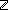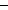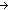represents the class of one-place integer-valued functions of integers; here's how:

For any (closed) expressions t1 and t2, t1 = t2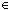just when

for any expressions r1 and r2, if r1 = r2then t1(r1) = t2(r2.

Specializing this condition variously,

if tand rthen t(rif tand r1 = r2then t(r1) = t(r2if t1 = t2and rthen t1(r) = t2(rGeneralizing away from domain and result types, the type AB represents the class of one-place B-valued functions of arguments from A. Here's how: t1 = t2AB just when

t1 and t2 execute giving result expressions "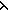x1.b1(x1)" and "x2.b2(x2)", and
for any expressions r1 and r2, if r1 = r2A then t1(r1) = t2(r2B.

This extra condition about the execution of t1 and t2 themselves evaluating to "x.<*>" forms is not theoretically essential, but it is standard. In the case ofabove we omitted mentioning it for simplicity, and because it follows from the way we happen to define evaluation of "f(r)" and the fact thathas members.

Note: We could have chosen to define AB without this condition on its members with the result that, when A was empty, every closed expression would denote the single degenerate member of AB. It is degenerate because it cannot be applied to anything in the domain A.

Identity on functions is extensional, consequently,

Thm*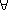f:(AB). (x.f(x)) = f

Thm*f,g:(AB). f = g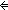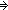(x:Af(x) = g(x))

Dependent Function Types: x:AC(x)

Actually, AB is a special form of the more general x:AC(x). When A is a type and C(x) is a type-valued function (in x) over domain A, then a member of x:AC(x) is a function which for an argument aA takes a value in the type C(a). That is, one can specify the type of the output as a function of the value of the input. So, t1 = t2x:AC(x) just when

t1 and t2 execute giving result expressions "x1.b1(x1)" and "x2.b2(x2)", and
for any expressions r1 and r2, if r1 = r2A then t1(r1) = t2(r2C(r1).

Since C(x) must be a type-valued function in xA, C(r1) and C(r2) must denote the same type, so we might as well have said "t1(r1) = t2(r2C(r2)" above.

This type constructor is also called the "general product" of a family of types, and indeed the most common notation for it is "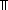x:AB(x)".

When the expression for the result type does not use the input variable, we get the special form for display AB.

(Sept 2001 - sfa )